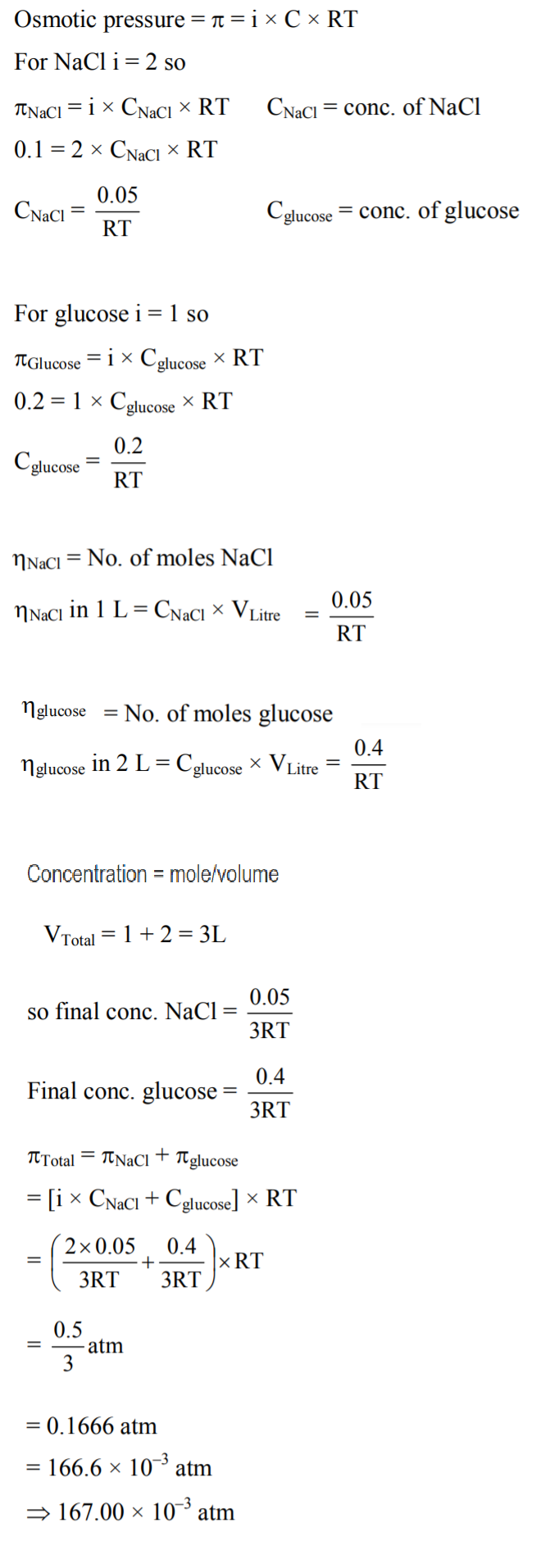Filter By

## All Questions

#### The freezing point of benzene decreases by 0.450C when 0.2 g of acetic acid is added to 20 g of benzene.  If acetic acid associates to form a dimer in benzene, percentage association of acetic acid in benzene will be : (Kf for benzene=5.12 K kg mol−1) Option: 1  74.6% Option: 2 94.6 Option: 3  64.6% Option: 4 80.4%

In benzene ,

t = 0                   1                                 -

t = t                      1 -

Given:

w = 0.2g                W = 20 g                  T = 0.45 K

As we know ,

, observed M = 113.78 (acetic acid)

Molecular weight of acetic acid = 60

% degree of association = 94.5 %

## Crack CUET with india's "Best Teachers"

• HD Video Lectures
• Unlimited Mock Tests
• Faculty Support#### At room temperature, a dilute solution of urea is prepared by dissolving 0.60g of urea in 360 g of water. If the vapour pressure of pure water at this temperature is 35 mmHg, lowering of vapour pressure(in  mmHg)  will be: (molar mass of urea = 60g/mol) Option: 1 0.017 Option: 2 0.028 Option: 3 0.027 Option: 4 0.031

Molecular weight of urea = 60 g / mol

Now, we know that :

Relative lowering of vapour pressure is equal to the molar mass of the solute.

option (1) is correct.

#### The elevation of boiling point of 0.1 m aq solution is two times that of 0.05 aq.   solution. The value of x _____ (Assume 100% ionization of complex and  , coordination number of Cr is 6 and that all of  molecules are present inside the coordination sphere.)

Thus, i = 3

Therefore, the complex is dissociated into 3 ions.

Hence, complex is

Thus, the value is 5.

## Crack NEET with "AI Coach"

• HD Video Lectures
• Unlimited Mock Tests
• Faculty Support#### A set of solutions is prepared using 180 g of water as a solvent and 10 g of different non-volatile solutes A,B and C. The relative lowring of vapour pressure in the presence of these solutes are in the order [Given, molar mass of A=100 g mol -1; B=200 g mol -1; C=10,000 g mol -1]Option: 1Option: 2Option: 3Option: 4Here vapour pressure of water is lowering.

We know this formula,

Partial Vapour pressure of water Pwater = PoXwater

Lowering Vapour pressure of water = Po – Pwater

The relative lowering of vapour pressure

Now,

From above,

## Crack JEE Main with "AI Coach"

• HD Video Lectures
• Unlimited Mock Tests
• Faculty Support#### The osmotic pressure of a solution of NaCl is 0.10 atm and that of a glucose solution is 0.20 atm. The osmotic pressure of a solution formed by mixing 1L of the sodium chloride solution 2L of the glucose solution is. x is _________ (nearest interger)

We know this formula.

Ans = 167

------------------------------------------

Alternate SolutionAns = 167

#### The mole fraction of glucose   in an aqueous binary solution is 0.1. The mass percentage of water in it to the nearest integer is-

Mole fraction of glucose, in

Now, lets assume we have 10 moles of solution.

Thus, moles of

Ans, moles of

Now, the mass of water = number of moles x molar mass

Thus, the mass of water

Mass of

Now, mass percent of water is given as:

Thus, the correct answer is 47.

## Crack CUET with india's "Best Teachers"

• HD Video Lectures
• Unlimited Mock Tests
• Faculty Support#### If  of an aqueous solution containing  of a protein A is isotonic with one litre of another aqueous solution containing of a protein , AT , the ratio of the molecular masses of and is ______ (to the nearest integer).

Let molar mass of protein A = x g/mol
Let molar mass of protein B = y g/mol

Now,

Ans = 177

#### 2 molal solution of a weak acid HA has a freezing point of 3.885oC. The degree of dissociation of this acid is _________(Round off to the Nearest Integer). [Given : Molal depression constant of waterFreezing point of pure water = 0o C]

Given,

2 molal solution of a weak acid HA has a freezing point of 3.885ºC.

m = 2

Molal depression constant of water (Kf) = 1.85 K kg mol-1

The freezing point of pure water  Ti = 0ºC

van't Hoff factor i is related to the degree of dissociation \alpha  as, if n = number of ions,

Here n = 2 (H+and A-

So,

We know the formula of depression at the freezing point.

i = 1.05

Now,

Ans = 50